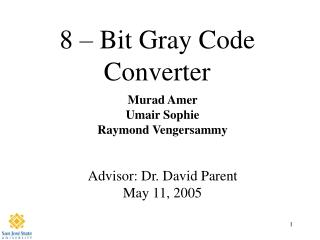DownloadDownload Presentation8 – Bit Gray Code Converter

# 8 – Bit Gray Code Converter

Download Presentation## 8 – Bit Gray Code Converter

- - - - - - - - - - - - - - - - - - - - - - - - - - - E N D - - - - - - - - - - - - - - - - - - - - - - - - - - -
##### Presentation Transcript

1. 8 – Bit Gray Code Converter Murad Amer Umair Sophie Raymond Vengersammy Advisor: Dr. David Parent May 11, 2005

2. Agenda • Abstract • Introduction • Why 8-bit gray code? • Theory of 8-bit gray code encoder • Background Information • Summary of Results • Project (Experimental) Details • Results • Cost Analysis • Conclusions

3. Abstract • 8 bit Gray Code Converter converts regular binary number to Gray code numbers • Binary  Gray • Operates at a clock frequency of 200 MHz • Power: 6mW • Area: 265mm x 150mm • DFF MS-Latch: Wn = 1.50, 1.65um, Wp = 2.55, 2.70 um SL-Latch: Wn = 1.95, 1.65 um, Wp = 1.65, 2.70 um • XOR AOI: Wn = 1.5um, Wp = 2.7um INV: Wn = 1.5um, Wp = 2.7um

4. Introduction Why the Binary Gray Code Converter??? • First of all, it applies many of the concepts from the class and lecture (EE-166) • BGCC, is applied in many applications • This project converts a series of binary numbers to gray code numbers with the use of XOR gates and DFF’s

5. Project Summary • Picked an initial load capacitance (Cload) • Partitioned the circuit into different propagation delay times according to gate/device requirements • Created the schematic, symbol and layout for each type of gate (XOR, MUX-DFF) • Limits the amount of error that can occur when several bits change between numbers

6. Conversion • Decimal Binary Gray Code 0 0000 0000 1 0001 0001 2 0010 0011 3 0011 0010

7. Project Details • Binary to Gray • Uniform cell heights of 30 μm • 7 XOR gates • 16 Mux based D-Flip Flops

8. Longest Path Calculations Note: All widths are in microns and capacitances in fF

9. Schematic (DFF)

10. Layout ( DFF)

11. Simulations (DFF)

12. Schematic (XOR)

13. Layout ( XOR)

14. Gray Code Schematic

15. Gray Code Layout

16. Verification

17. Gray Code Simulation

18. Cost Analysis • We Spent Many Hours on this Project • Verifying logic = 10 hrs • Verifying timing = 30 hrs • Layout = 25 hrs • Post extracted timing = 5 hrs

19. Lessons Learned • START EARLY! • FOCUS in class • START EARLY!! • Utilize other students in the class • START EARLY!!! • Work as a TEAM efficiently • START EARLY!!!!

20. Summary • This Project explained the Fundamentals of EE-166 • Taught us the Ins - and - Outs of basic Design • Less Power Used, Less Area Used • Met Specifications

21. Acknowledgements • Thanks to our families for putting up with us for not being home. • Thanks to Cadence Design Systems for the VLSI lab • Thanks to Synopsys for Software donation • Dr. David Parent • Thanks to the janitors/security for letting us spend late hours in the labs.